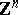Every irreducible representation is indecomposable. The class of finite-dimensional indecomposable representations of the groupand the decomposition of a given finite-dimensional representation ofinto indecomposable ones are directly connected with the Jordan normal form of a matrix and the theory of linear ordinary differential equations with constant coefficients. The classification of indecomposable representations of even such groups asand,, is (1982) far from complete. Indecomposable representations of semi-direct products of groups, in particular, of solvable Lie groups, can be reducible (even in the finite-dimensional case). On the other hand, finite-dimensional indecomposable representations of real semi-simple Lie groups are irreducible. However, these groups have reducible infinite-dimensional indecomposable representations, notably, the analytic continuation of the fundamental continuous series of representations of such groups.